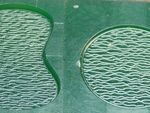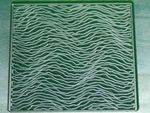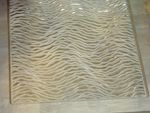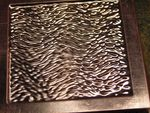﻿ Procedural texture in Rhino# Procedural texture in Rhino (02/12/13)

In the background of lots of our jewellery designs, I use either a linear or radial "clearout" toolpath. See, for example, this pendant:

The background shading in that design was done with lines 0.25mm apart using a 0.5mm ball-nose cutter. The linear shading pattern than results is very nice, but I realised that some pieces might benefit from a more random texture.

All our design work is done in Rhino. This comes with a very powerful scripting language called Rhinoscript, which is based on VBScript. I haven't used it much, but it can automate any of the tasks which are normally performed by the user. For example, I used it to do some basic animation.

I whipped up a little script to create a series of control point curves through sets of points which had a slight random variation added to them - this resulted in nice lines which were predominantly parallel and straight, but with slight random wiggles. Here's the code (you can download the script here):

```' 20/11/13: for 0.5mm ballnose

Sub drawWavy()

' Definitions
Dim arrPoints(50)

' Delete everything
allobj=Rhino.allobjects
If IsArray(allobj) Then Rhino.deleteobjects allobj

' Draw lines
For y=0 To 50

' Load point array with data
For x=0 To 50
arrPoints(x)=Array((x+((Rnd()-0.5)*.5))/3,(y+((Rnd()-0.5)*1))/3,0)
Next

' Draw the control points
Rhino.currentlayer("Layer 01")
Rhino.currentlayer("Default")

' Draw control point curve

' Slow things down so user can see it drawing
Rhino.sleep 100

Next
End Sub

' Run the sub
drawWavy()```

For each Y position from 0 to 50, it creates an array of 50 points in the horizontal direction with random variations in both their X " Y coordinates and then draws a control point curve through them.

By simply varying how the program calculates the points, it's possible to create some interesting effects. For example, by using

`arrPoints(x)=Array((x+((Rnd()-0.5)*.5))/3,((y+((Rnd()-0.5)*1))/3)+(0.5*Sin((x/3)-(Sin(y/5)))),0)`"Straight" linesWavy linesStraight lines in modelling waxWavy lines in modelling waxWavy lines in acrylicCopper foil pressed into patternReverse side